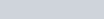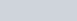Find the centre of the circle passing through
Question:

Find the centre of the circle passing through (6, −6), (3, −7) and (3, 3).

Solution:

The distance $d$ between two points $\left(x_{1}, y_{1}\right)$ and $\left(x_{2}, y_{2}\right)$ is given by the formula

$d=\sqrt{\left(x_{1}-x_{2}\right)^{2}+\left(y_{1}-y_{2}\right)^{2}}$

The centre of a circle is at equal distance from all the points on its circumference.

Here it is given that the circle passes through the points A(6,6), B(3,7) and C(3,3).

Let the centre of the circle be represented by the point O(x, y).

So we have$A O=\sqrt{(6-x)^{2}+(-6-y)^{2}}$

$B O=\sqrt{(3-x)^{2}+(-7-y)^{2}}$

$C O=\sqrt{(3-x)^{2}+(3-y)^{2}}$

Equating the first pair of these equations we have,

$A O=B O$

$\sqrt{(6-x)^{2}+(-6-y)^{2}}=\sqrt{(3-x)^{2}+(-7-y)^{2}}$

Squaring on both sides of the equation we have,

$(6-x)^{2}+(-6-y)^{2}=(3-x)^{2}+(-7-y)^{2}$

$36+x^{2}-12 x+36+y^{2}+12 y=9+x^{2}-6 x+49+y^{2}+14 y$

$6 x+2 y=14$

$3 x+y=7$

Equating another pair of the equations we have,

$A O=C O$

$\sqrt{(6-x)^{2}+(-6-y)^{2}}=\sqrt{(3-x)^{2}+(3-y)^{2}}$

Squaring on both sides of the equation we have,

$(6-x)^{2}+(-6-y)^{2}=(3-x)^{2}+(3-y)^{2}$

$36+x^{2}-12 x+36+y^{2}+12 y=9+x^{2}-6 x+9+y^{2}-6 y$

$6 x-18 y=54$

$x-3 y=9$

Now we have two equations for ‘x’ and ‘y’, which are

$3 x+y=7$

$x-3 y=9$

From the second equation we have. Substituting this value of ‘y’ in the first equation we have,

$x-3(-3 x+7)=9$

$x+9 x-21=9$

$10 x=30$

$x=3$

Therefore the value of ‘y’ is,

$y=-3 x+7$

$=-3(3)+7$

$y=-2$

Hence the co-ordinates of the centre of the circle are $(3,-2)$.